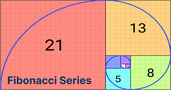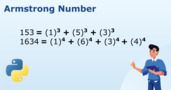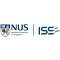What is a Rational Number?

# What is a Rational Number?

clickHere
Updated on Sep 27, 2023 17:19 IST

Learn about rational numbers, their characteristics and types. Understand the concept of rational numbers and their applications through mathematical examples.

Rational Number Definition – A rational number can be expressed as a ratio of two integers. They are categorized under Real Numbers.

Content

Rational numbers can be represented as a/b, where a is the numerator, b is the denominator, and b ≠ 0. Suppose a = 2 and b = 4; then its representation would be –

## Rational Number Analogy

Imagine you have a pizza. You can share that pizza with your friends. If you cut the pizza into equal pieces and have some of those pieces, like 1 piece or 2 pieces or even 3 pieces, those are like rational numbers.
A rational number is a fancy way of saying a number that can be made by dividing one whole number by another. The top number is called the ‘numerator’ (pieces of pizza you have), and the bottom number is called the ‘denominator’ (the number of parts the whole pizza is divided into).

So, if you have 1/2 of the pizza, that’s a rational number because you took 1 piece out of 2 equal pieces. If you have 3/4 of the pizza, that’s also rational because you took 3 out of 4 equal pieces.
But, if you have a strange number like the square root of 2 (about 1.41421356…), you can’t make it by dividing whole numbers, so it’s not rational. It’s called an ‘irrational’ number.
So, in simple words, a rational number is like a piece of pizza that you can get by dividing a pizza into equal parts, and both the top and bottom numbers are whole numbers.

In other words, rational numbers are real numbers that can be rewritten as the ratio of two integers because both the numerator and denominator are known.Factorial Programs in C – A Step-by-Step Guide
In this article, we will discuss how to calculate the factorial programming in c using three different methods: For Loops, Recursion, and While Loop.How to Find the Factorial of a Number Using Python
In this article, we will discuss different methods to find the factorial of a number in python using for, while, ternary operator and math module.Number Functions in Tableau: Mathematical, Trigonometric
In this article, we will discuss number functions in tableau like mathematical and trigonometric function.

## Characteristics of Rational Numbers

• They are countable
• Can be expressed as terminating Decimal Numbers (2.5) and non-terminating repeating decimal numbers (0.2323232323…)
• Between two rational numbers, there are infinitely many rational numbers
• Rational numbers are a superset that contains the integers, whole, and natural numbers

You May Like – What is a Prime Number?

## Types of Rational Numbers

The real numbers are divided into irrational and rational numbers. And rational numbers can be reduced to integers, whole, and natural numbers. There are two main types of rational numbers: integers and natural numbers.

The different types of rational numbers are:

• Integers like -4, -2, 0, 3 etc.
• Fractions where numerators and denominators are integers like 2/7, -4/5, etc.
• Terminating decimals like 0.35, 0.7116, 0.9768, etc.
• Non-terminating decimals with some repeating patterns after the decimal. Examples include 0.7777…, 0.6666667…, 0.141414…, etc. These types of number strings are non-terminating repeating decimals.

Let us understand the above types of rational numbers through examples.Fibonacci Number
In this article, we will discuss Fibonacci numbers, it’s application and how to generate them using Python, Java and C.Different Methods to Check for Prime Numbers in Python
As a Python beginner, when you are practising coding, one of the most common programs you will work on is determining whether a number is prime or not.How to Find an Armstrong Number Using Python
If a positive integer is equal to the sum of each digit powered with the total number of digits, then that number is called Armstrong Number. In this article, we...read more

## Rational Numbers Examples

There are infinite numbers so that we can make infinite fractions of whole numbers. However, we must know how to differentiate when a number is irrational.

### Example 1

For example –

Is 8.75 a rational number?

Yes, because we can express it as a fraction:

### Example 2

Example of a rational number

Is 2.718281828459047… a rational number?

No, because we cannot express it as a fraction:

### Example 3

Example of an irrational number

Is 5.666666666666667 a rational number?

Yes, even though there are decimals and the series continues to infinity, it is a fraction:

### Example 4

An example of a rational number

An interesting point to discuss is – Are all roots rational numbers?

The answer is that some roots are rational numbers, and some are irrational. For example, the square root of four is a rational number, but the square root of 93 is irrational.

Interesting Read – How to Find the Factorial of a Number Using Python

## Is Pi A Rational Number?

Pi is a mathematical expression. It has an approximate value is 3.14159365…

You can see that the value of π is expressed in decimal, which is non-terminating and non-repeating. Since the value of Pi is non-terminating, it is an irrational number.

Hence, Pi is not a rational number.

## How to Identify if a Number is Rational?

Numbers in the form of numerators and denominators are rational numbers, but if the unknown is a decimal, we must check if it is a rational or irrational. Check if a number or a set of numbers repeats in the decimal part of a number group. Below is the classification of these numbers.

• 0.857142857142857142857142 ⇒ Periodical = 857142 ← Periodical Pure
• 2.8 ⇒ Restricted
• 2.08333333333 ⇒ Periodical = 83 ← Periodical Mixed

Suppose you have an unknown as a decimal number, such as

• 0.243434343434…
• 123.187534883320…
• 6.01001000100001…

In such cases, we will check if the numbers repeat.

• Rational, Periodical = 34, Periodical Mixed
• Rational, Periodical = 187534, Periodical Pure
• Irrational

If there is repetition after a certain number, we can call it rational, but if there is no defined period, we can call it an irrational number.

Related Read – Different Methods to Check for Prime Numbers in Python

## Arithmetic Operations on Rational Numbers

Let us check how we can perform arithmetic operations on rational numbers, for example –

a/b and x/y.

Addition: To add a/b and x/y; we will use the following formula –

(ay+bx)/by.

Example: 1/4 + 4/5 ⇒ (1×5)+(4×4)/20 ⇒ 5+16/20 ⇒ 21/20

Subtraction: Similarly, when subtracting a/b and x/y; we will use the formula –

(ay-bx)/by

Example: 1/2 – 3/4 = (2-3)/4 = -1/4

3/4  – 1/3 ⇒ (3×3) – (4X1)/4X3 = 9 – 4/12 ⇒ 5/12

Multiplication: If a/b multiplies by x/y, then we get (a × x)/(b x y).

Example: 1/4 × 2/3 ⇒ (1×2)/(4×3) ⇒ 2/12 ⇒ 1/6

Division: If a/b is divided by x/y, then it is represented as:

(a/b)÷(x/y) = ay/bx

Example: 1/3 ÷ 4/5 = (1×5)/(3×4) = 5/12

## C++ Program For Adding Rational Numbers

` `
```// Finding the sum of two fractions#include <iostream>using namespace std;int main() {    // Write C++ code here    float num_1, num_2, den_1, den_2;    cout << "Enter the first fraction numerator and denominator";    cin>>num_1;    cin>>den_1;    cout << "Enter the second fraction numerator and denominator";    cin>>num_2;    cin>>den_2;     float sum;    sum = (num_1*den_2 + num_2*den_1)/(den_1*den_2);    cout<<sum;     return 0;}Copy code```

Output:

In a Nutshell

Rational numbers are all those that a mathematical fraction can express. We hope this article helped you understand the concept of rational numbers through examples.

## FAQs

What is a rational number in math?

Any number that can be written as a ratio or fraction of two integers is rational.

What is an example of a rational number?

A rational number exists in the form of p/q where q not equal to 0. Any fraction that has non-zero denominators is a rational number. Some examples of rational numbers are 1/2, 1/4, 5/4, and so on.

Is two a rational number?

Yes, 2 is a rational number. It satisfies the condition for rational number, and we can write it in the p/q form, represented as 2/1.

How do you know if a number is rational?

A rational number can be written as a ratio or as a fraction. In such numbers, both the numerator and the denominator are whole numbers. The number 8 is rational because we can write it as a fraction, 8/1.

Which are irrational numbers?

An irrational number is a type of real number that cannot be represented as a simple fraction, i.e., if N is an irrational, then N cannot be expressed in the form of p/q where p and q are integers and q is not equal to 0. Example: pi, e (Euler number), 0.3435687697......

Is zero an irrational number?

No, zero is a rational number since, it can be expressed as 0/1 (i.e., p/q form).

clickHere

Rashmi is a postgraduate in Biotechnology with a flair for research-oriented work and has an experience of over 13 years in content creation and social media handling. She has a diversified writing portfolio and aim... Read Full Bio

## Trending Data Science CoursesBecome a Data Science Professional with IIT Certification in Advanced ProgrammingBecome a Data Science Professional with IIT Certif...
Guvi5.0AI For Everyone
Coursera4.5Data Science with Python
UDEMY4.2Postgraduate Program in Data Science with Generative AIPostgraduate Program in Data Science with Generati...
Praxis Tech School4.8Starts 5 DecBecome a Data Science Professional with IIT Certification in Advanced ProgrammingBecome a Data Science Professional with IIT Certif...
Guvi5.0Starts 5 Dec## Top Picks & New Arrivals